### 4.0.3 is buuugggggggyyyyyy

#### 2DIndie

Wow, i'm new to stencyl, but 4.0.3 is has a lot of bugs.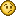It's not the first time stencyl crashed me!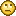As i saw stencyl i was thinking wow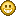, that's a good game engine... but at this moment i don't know.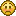issue:
I've opend my game, added a tileset (normal 16x16) with a "wrong size" (13x16) and now i can't deletethis tileset because
a) it's hidden ( i don't see it)
b) stencyl crashed in this "unexpected Problem" if i go to "Resources - Tileset"

So only killing stencyl with taskmanager works.
Open game again and go to "Resources - Tileset" crashed again in the same issue - this is a loop!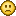#### merrak

Code: [Select]
`an't read input file! for file C:\Users\2DIndie1\AppData\Roaming\Stencyl\stencylworks\games\SunnyLand Forest\resources\9.png2020-09-07 19:06:01,942 ERROR [AWT-EventQueue-0] root: Unexpected problem on thread AWT-EventQueue-0: Width (78) and height (0) cannot be <= 0`
This image file looks like your problem. It somehow got saved with a height of 0. You can probably just delete that file and replace it with the intended tileset image. I'd suggest filing a report in the issue tracker as well.

#### 2DIndie

In that path there is no file named 9.png.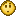I would like to use the issue tracker but i can't find the version 4.0.3 to choose.#### 2DIndie

So, if anybody can help me to open my game so i can work on it... it would be nice.
I've to fix this "unexpected Problem" first!

#### 2DIndie

If i can't open my game to work on it i've to quit working with stencyl.Is there no way i can fix this problem?#### yoplalala

Copy your game somewhere ( as a backup) .

Go to your game folder , goto  the folder "resources".

open data.xml
Look for somthing like this

Code: [Select]
`   <actor adamp="0.0" ascale="true" atlasID="0" bodytype="2" continuous="true" desc="" eventsnippetid="31" fixedrot="false" fric="0.2" gid="3" id="75" ignoreg="false" inertia="1.0" ldamp="0.0" mass="1.0" name="languageIcon" oid="-1" pausable="true" physicsMode="0" rest="0.0" shared="false" sprite="76" static="false">        <snippets/>    </actor>` where sprite="9"

and something like this

Code: [Select]
`   <sprite atlasID="0" default="0" defaultAnimation="0" desc="" id="76" name="languageIcon" readable="false" shared="false">        <anim across="1" down="1" durations="100" height="48" id="0" loop="true" name="Animation 0" numframes="1" originx="0" originy="0" sync="false" version="2" width="48"/>    </sprite>`where id="9"

I've never had this problem so I'm not 100 % sur it will work but logically it should. (if it's th case , you can post your broken game and I'll look at it)

#### 2DIndie

Thx, have make a .zip file from this folder to backup the game.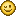Sorry but there is no sprite="9" in it.Code: [Select]
`<?xml version="1.0" encoding="UTF-8" standalone="no"?><pack>    <background atlasID="0" desc="" durations="100" dx="-0.0" dy="-0.0" height="240" id="3" name="bg" numframes="1" oid="-1" readable="false" repeats="true" resized="false" shared="false" width="192" xpf="1.0" ypf="1.0"/>    <tileset across="20" atlasID="0" desc="" down="12" id="0" name="layers" oid="-1" readable="false" shared="false" th="16" tw="16">        <tile collision="0" data="" durations="100" frames="0" id="1" order="-1"/>        <tile collision="0" data="" durations="100" frames="1" id="3" order="-1"/>        <tile collision="0" data="" durations="100" frames="2" id="4" order="-1"/>        <tile collision="0" data="" durations="100" frames="3" id="5" order="-1"/>        <tile collision="0" data="" durations="100" frames="4" id="6" order="-1"/>        <tile collision="0" data="" durations="100" frames="5" id="8" order="-1"/>        <tile collision="0" data="" durations="100" frames="6" id="10" order="-1"/>        <tile collision="0" data="" durations="100" frames="7" id="12" order="-1"/>        <tile collision="0" data="" durations="100" frames="8" id="2" order="-1"/>        <tile collision="0" data="" durations="100" frames="9" id="29" order="-1"/>        <tile collision="0" data="" durations="100" frames="10" id="7" order="-1"/>        <tile collision="0" data="" durations="100" frames="11" id="11" order="-1"/>        <tile collision="0" data="" durations="100" frames="12" id="9" order="-1"/>        <tile collision="0" data="" durations="100" frames="13" id="13" order="-1"/>        <tile collision="0" data="" durations="100" frames="14" id="14" order="-1"/>        <tile collision="0" data="" durations="100" frames="15" id="15" order="-1"/>        <tile collision="0" data="" durations="100" frames="16" id="16" order="-1"/>        <tile collision="0" data="" durations="100" frames="17" id="17" order="-1"/>        <tile collision="0" data="" durations="100" frames="18" id="18" order="-1"/>        <tile collision="0" data="" durations="100" frames="19" id="19" order="-1"/>        <tile collision="0" data="" durations="100" frames="20" id="20" order="-1"/>        <tile collision="0" data="" durations="100" frames="21" id="23" order="-1"/>        <tile collision="0" data="" durations="100" frames="22" id="22" order="-1"/>        <tile collision="0" data="" durations="100" frames="23" id="21" order="-1"/>        <tile collision="0" data="" durations="100" frames="24" id="24" order="-1"/>        <tile collision="0" data="" durations="100" frames="25" id="25" order="-1"/>        <tile collision="0" data="" durations="100" frames="26" id="0" order="-1"/>        <tile collision="0" data="" durations="100" frames="27" id="27" order="-1"/>        <tile collision="0" data="" durations="100" frames="28" id="28" order="-1"/>        <tile collision="0" data="" durations="100" frames="29" id="26" order="-1"/>        <tile collision="0" data="" durations="100" frames="30" id="30" order="-1"/>        <tile collision="0" data="" durations="100" frames="31" id="31" order="-1"/>        <tile collision="0" data="" durations="100" frames="32" id="32" order="-1"/>        <tile collision="0" data="" durations="100" frames="33" id="33" order="-1"/>        <tile collision="0" data="" durations="100" frames="34" id="34" order="-1"/>        <tile collision="0" data="" durations="100" frames="35" id="35" order="-1"/>        <tile collision="0" data="" durations="100" frames="36" id="36" order="-1"/>        <tile collision="0" data="" durations="100" frames="37" id="37" order="-1"/>        <tile collision="0" data="" durations="100" frames="38" id="38" order="-1"/>        <tile collision="0" data="" durations="100" frames="39" id="39" order="-1"/>        <tile collision="0" data="" durations="100" frames="40" id="40" order="-1"/>        <tile collision="0" data="" durations="100" frames="41" id="41" order="-1"/>        <tile collision="0" data="" durations="100" frames="42" id="42" order="-1"/>        <tile collision="0" data="" durations="100" frames="43" id="43" order="-1"/>        <tile collision="0" data="" durations="100" frames="44" id="44" order="-1"/>        <tile collision="0" data="" durations="100" frames="45" id="45" order="-1"/>        <tile collision="0" data="" durations="100" frames="46" id="46" order="-1"/>        <tile collision="0" data="" durations="100" frames="47" id="47" order="-1"/>        <tile collision="0" data="" durations="100" frames="48" id="48" order="-1"/>        <tile collision="0" data="" durations="100" frames="49" id="49" order="-1"/>        <tile collision="0" data="" durations="100" frames="50" id="50" order="-1"/>        <tile collision="0" data="" durations="100" frames="51" id="51" order="-1"/>        <tile collision="0" data="" durations="100" frames="52" id="52" order="-1"/>        <tile collision="0" data="" durations="100" frames="53" id="53" order="-1"/>        <tile collision="0" data="" durations="100" frames="54" id="54" order="-1"/>        <tile collision="0" data="" durations="100" frames="55" id="55" order="-1"/>        <tile collision="0" data="" durations="100" frames="56" id="56" order="-1"/>        <tile collision="0" data="" durations="100" frames="57" id="57" order="-1"/>        <tile collision="0" data="" durations="100" frames="58" id="58" order="-1"/>        <tile collision="0" data="" durations="100" frames="59" id="59" order="-1"/>        <tile collision="0" data="" durations="100" frames="60" id="60" order="-1"/>        <tile collision="0" data="" durations="100" frames="61" id="61" order="-1"/>        <tile collision="0" data="" durations="100" frames="62" id="62" order="-1"/>        <tile collision="0" data="" durations="100" frames="63" id="63" order="-1"/>        <tile collision="0" data="" durations="100" frames="64" id="64" order="-1"/>        <tile collision="0" data="" durations="100" frames="65" id="65" order="-1"/>        <tile collision="0" data="" durations="100" frames="66" id="66" order="-1"/>        <tile collision="0" data="" durations="100" frames="67" id="67" order="-1"/>        <tile collision="0" data="" durations="100" frames="68" id="68" order="-1"/>        <tile collision="0" data="" durations="100" frames="69" id="69" order="-1"/>        <tile collision="0" data="" durations="100" frames="70" id="70" order="-1"/>        <tile collision="0" data="" durations="100" frames="71" id="71" order="-1"/>        <tile collision="0" data="" durations="100" frames="72" id="72" order="-1"/>        <tile collision="0" data="" durations="100" frames="73" id="73" order="-1"/>        <tile collision="0" data="" durations="100" frames="74" id="74" order="-1"/>        <tile collision="0" data="" durations="100" frames="75" id="75" order="-1"/>        <tile collision="0" data="" durations="100" frames="76" id="76" order="-1"/>        <tile collision="0" data="" durations="100" frames="77" id="77" order="-1"/>        <tile collision="0" data="" durations="100" frames="78" id="78" order="-1"/>        <tile collision="0" data="" durations="100" frames="79" id="79" order="-1"/>        <tile collision="0" data="" durations="100" frames="80" id="80" order="-1"/>        <tile collision="0" data="" durations="100" frames="81" id="81" order="-1"/>        <tile collision="0" data="" durations="100" frames="82" id="82" order="-1"/>        <tile collision="0" data="" durations="100" frames="83" id="83" order="-1"/>        <tile collision="0" data="" durations="100" frames="84" id="84" order="-1"/>        <tile collision="0" data="" durations="100" frames="85" id="85" order="-1"/>        <tile collision="0" data="" durations="100" frames="86" id="86" order="-1"/>        <tile collision="0" data="" durations="100" frames="87" id="87" order="-1"/>        <tile collision="0" data="" durations="100" frames="88" id="88" order="-1"/>        <tile collision="0" data="" durations="100" frames="89" id="89" order="-1"/>        <tile collision="0" data="" durations="100" frames="90" id="90" order="-1"/>        <tile collision="-1" data="" durations="100" frames="91" id="91" order="-1"/>        <tile collision="0" data="" durations="100" frames="92" id="92" order="-1"/>        <tile collision="0" data="" durations="100" frames="93" id="93" order="-1"/>        <tile collision="0" data="" durations="100" frames="94" id="94" order="-1"/>        <tile collision="0" data="" durations="100" frames="95" id="95" order="-1"/>        <tile collision="0" data="" durations="100" frames="96" id="96" order="-1"/>        <tile collision="0" data="" durations="100" frames="97" id="97" order="-1"/>        <tile collision="0" data="" durations="100" frames="98" id="98" order="-1"/>        <tile collision="0" data="" durations="100" frames="99" id="99" order="-1"/>        <tile collision="0" data="" durations="100" frames="100" id="100" order="-1"/>        <tile collision="0" data="" durations="100" frames="101" id="101" order="-1"/>        <tile collision="0" data="" durations="100" frames="102" id="102" order="-1"/>        <tile collision="0" data="" durations="100" frames="103" id="103" order="-1"/>        <tile collision="0" data="" durations="100" frames="104" id="104" order="-1"/>        <tile collision="0" data="" durations="100" frames="105" id="105" order="-1"/>        <tile collision="0" data="" durations="100" frames="106" id="106" order="-1"/>        <tile collision="0" data="" durations="100" frames="107" id="107" order="-1"/>        <tile collision="0" data="" durations="100" frames="108" id="108" order="-1"/>        <tile collision="0" data="" durations="100" frames="109" id="109" order="-1"/>        <tile collision="0" data="" durations="100" frames="110" id="110" order="-1"/>        <tile collision="0" data="" durations="100" frames="111" id="111" order="-1"/>        <tile collision="0" data="" durations="100" frames="112" id="112" order="-1"/>        <tile collision="0" data="" durations="100" frames="113" id="113" order="-1"/>        <tile collision="0" data="" durations="100" frames="114" id="114" order="-1"/>        <tile collision="0" data="" durations="100" frames="115" id="115" order="-1"/>        <tile collision="0" data="" durations="100" frames="116" id="116" order="-1"/>        <tile collision="0" data="" durations="100" frames="117" id="117" order="-1"/>        <tile collision="0" data="" durations="100" frames="118" id="118" order="-1"/>        <tile collision="0" data="" durations="100" frames="119" id="119" order="-1"/>        <tile collision="0" data="" durations="100" frames="120" id="120" order="-1"/>        <tile collision="0" data="" durations="100" frames="121" id="121" order="-1"/>        <tile collision="0" data="" durations="100" frames="122" id="122" order="-1"/>        <tile collision="0" data="" durations="100" frames="123" id="123" order="-1"/>        <tile collision="0" data="" durations="100" frames="124" id="124" order="-1"/>        <tile collision="0" data="" durations="100" frames="125" id="125" order="-1"/>        <tile collision="0" data="" durations="100" frames="126" id="126" order="-1"/>        <tile collision="0" data="" durations="100" frames="127" id="127" order="-1"/>        <tile collision="0" data="" durations="100" frames="128" id="128" order="-1"/>        <tile collision="0" data="" durations="100" frames="129" id="129" order="-1"/>        <tile collision="0" data="" durations="100" frames="130" id="130" order="-1"/>        <tile collision="0" data="" durations="100" frames="131" id="131" order="-1"/>        <tile collision="0" data="" durations="100" frames="132" id="132" order="-1"/>        <tile collision="0" data="" durations="100" frames="133" id="133" order="-1"/>        <tile collision="0" data="" durations="100" frames="134" id="134" order="-1"/>        <tile collision="0" data="" durations="100" frames="135" id="135" order="-1"/>        <tile collision="0" data="" durations="100" frames="136" id="136" order="-1"/>        <tile collision="0" data="" durations="100" frames="137" id="137" order="-1"/>        <tile collision="0" data="" durations="100" frames="138" id="138" order="-1"/>        <tile collision="0" data="" durations="100" frames="139" id="139" order="-1"/>        <tile collision="0" data="" durations="100" frames="140" id="140" order="-1"/>        <tile collision="0" data="" durations="100" frames="141" id="141" order="-1"/>        <tile collision="0" data="" durations="100" frames="142" id="142" order="-1"/>        <tile collision="0" data="" durations="100" frames="143" id="143" order="-1"/>        <tile collision="0" data="" durations="100" frames="144" id="144" order="-1"/>        <tile collision="0" data="" durations="100" frames="145" id="145" order="-1"/>        <tile collision="0" data="" durations="100" frames="146" id="146" order="-1"/>        <tile collision="0" data="" durations="100" frames="147" id="147" order="-1"/>        <tile collision="0" data="" durations="100" frames="148" id="148" order="-1"/>        <tile collision="0" data="" durations="100" frames="149" id="149" order="-1"/>        <tile collision="0" data="" durations="100" frames="150" id="150" order="-1"/>        <tile collision="0" data="" durations="100" frames="151" id="151" order="-1"/>        <tile collision="0" data="" durations="100" frames="152" id="152" order="-1"/>        <tile collision="0" data="" durations="100" frames="153" id="153" order="-1"/>        <tile collision="0" data="" durations="100" frames="154" id="154" order="-1"/>        <tile collision="0" data="" durations="100" frames="155" id="155" order="-1"/>        <tile collision="0" data="" durations="100" frames="156" id="156" order="-1"/>        <tile collision="0" data="" durations="100" frames="157" id="157" order="-1"/>        <tile collision="0" data="" durations="100" frames="158" id="158" order="-1"/>        <tile collision="0" data="" durations="100" frames="159" id="159" order="-1"/>        <tile collision="0" data="" durations="100" frames="160" id="160" order="-1"/>        <tile collision="0" data="" durations="100" frames="161" id="161" order="-1"/>        <tile collision="0" data="" durations="100" frames="162" id="162" order="-1"/>        <tile collision="0" data="" durations="100" frames="163" id="163" order="-1"/>        <tile collision="0" data="" durations="100" frames="164" id="164" order="-1"/>        <tile collision="0" data="" durations="100" frames="165" id="165" order="-1"/>        <tile collision="0" data="" durations="100" frames="166" id="166" order="-1"/>        <tile collision="0" data="" durations="100" frames="167" id="167" order="-1"/>        <tile collision="0" data="" durations="100" frames="168" id="168" order="-1"/>        <tile collision="0" data="" durations="100" frames="169" id="169" order="-1"/>        <tile collision="0" data="" durations="100" frames="170" id="170" order="-1"/>        <tile collision="0" data="" durations="100" frames="171" id="171" order="-1"/>        <tile collision="0" data="" durations="100" frames="172" id="172" order="-1"/>        <tile collision="0" data="" durations="100" frames="173" id="173" order="-1"/>        <tile collision="0" data="" durations="100" frames="174" id="174" order="-1"/>        <tile collision="0" data="" durations="100" frames="175" id="175" order="-1"/>        <tile collision="0" data="" durations="100" frames="176" id="176" order="-1"/>        <tile collision="0" data="" durations="100" frames="177" id="177" order="-1"/>        <tile collision="0" data="" durations="100" frames="178" id="178" order="-1"/>        <tile collision="0" data="" durations="100" frames="179" id="179" order="-1"/>        <tile collision="0" data="" durations="100" frames="180" id="180" order="-1"/>        <tile collision="0" data="" durations="100" frames="181" id="181" order="-1"/>        <tile collision="0" data="" durations="100" frames="182" id="182" order="-1"/>        <tile collision="0" data="" durations="100" frames="183" id="183" order="-1"/>        <tile collision="0" data="" durations="100" frames="184" id="184" order="-1"/>        <tile collision="0" data="" durations="100" frames="185" id="185" order="-1"/>        <tile collision="0" data="" durations="100" frames="186" id="186" order="-1"/>        <tile collision="0" data="" durations="100" frames="187" id="187" order="-1"/>        <tile collision="0" data="" durations="100" frames="188" id="188" order="-1"/>        <tile collision="0" data="" durations="100" frames="189" id="189" order="-1"/>        <tile collision="0" data="" durations="100" frames="190" id="190" order="-1"/>        <tile collision="0" data="" durations="100" frames="191" id="191" order="-1"/>        <tile collision="0" data="" durations="100" frames="192" id="192" order="-1"/>        <tile collision="0" data="" durations="100" frames="193" id="193" order="-1"/>        <tile collision="0" data="" durations="100" frames="194" id="194" order="-1"/>        <tile collision="0" data="" durations="100" frames="195" id="195" order="-1"/>        <tile collision="0" data="" durations="100" frames="196" id="196" order="-1"/>        <tile collision="0" data="" durations="100" frames="197" id="197" order="-1"/>        <tile collision="0" data="" durations="100" frames="198" id="198" order="-1"/>        <tile collision="0" data="" durations="100" frames="199" id="199" order="-1"/>        <tile collision="0" data="" durations="100" frames="200" id="200" order="-1"/>        <tile collision="0" data="" durations="100" frames="201" id="201" order="-1"/>        <tile collision="0" data="" durations="100" frames="202" id="202" order="-1"/>        <tile collision="0" data="" durations="100" frames="203" id="203" order="-1"/>        <tile collision="0" data="" durations="100" frames="204" id="204" order="-1"/>        <tile collision="0" data="" durations="100" frames="205" id="205" order="-1"/>        <tile collision="0" data="" durations="100" frames="206" id="206" order="-1"/>        <tile collision="0" data="" durations="100" frames="207" id="207" order="-1"/>        <tile collision="0" data="" durations="100" frames="208" id="208" order="-1"/>        <tile collision="0" data="" durations="100" frames="209" id="209" order="-1"/>        <tile collision="0" data="" durations="100" frames="210" id="210" order="-1"/>        <tile collision="0" data="" durations="100" frames="211" id="211" order="-1"/>        <tile collision="0" data="" durations="100" frames="212" id="212" order="-1"/>        <tile collision="0" data="" durations="100" frames="213" id="213" order="-1"/>        <tile collision="0" data="" durations="100" frames="214" id="214" order="-1"/>        <tile collision="0" data="" durations="100" frames="215" id="215" order="-1"/>        <tile collision="0" data="" durations="100" frames="216" id="216" order="-1"/>        <tile collision="0" data="" durations="100" frames="217" id="217" order="-1"/>        <tile collision="0" data="" durations="100" frames="218" id="218" order="-1"/>        <tile collision="0" data="" durations="100" frames="219" id="219" order="-1"/>        <tile collision="0" data="" durations="100" frames="220" id="220" order="-1"/>        <tile collision="0" data="" durations="100" frames="221" id="221" order="-1"/>        <tile collision="0" data="" durations="100" frames="222" id="222" order="-1"/>        <tile collision="0" data="" durations="100" frames="223" id="223" order="-1"/>        <tile collision="0" data="" durations="100" frames="224" id="224" order="-1"/>        <tile collision="0" data="" durations="100" frames="225" id="225" order="-1"/>        <tile collision="0" data="" durations="100" frames="226" id="226" order="-1"/>        <tile collision="0" data="" durations="100" frames="227" id="227" order="-1"/>        <tile collision="0" data="" durations="100" frames="228" id="228" order="-1"/>        <tile collision="0" data="" durations="100" frames="229" id="229" order="-1"/>        <tile collision="0" data="" durations="100" frames="230" id="230" order="-1"/>        <tile collision="0" data="" durations="100" frames="231" id="231" order="-1"/>        <tile collision="0" data="" durations="100" frames="232" id="232" order="-1"/>        <tile collision="0" data="" durations="100" frames="233" id="233" order="-1"/>        <tile collision="0" data="" durations="100" frames="234" id="234" order="-1"/>        <tile collision="0" data="" durations="100" frames="235" id="235" order="-1"/>        <tile collision="0" data="" durations="100" frames="236" id="236" order="-1"/>        <tile collision="0" data="" durations="100" frames="237" id="237" order="-1"/>        <tile collision="0" data="" durations="100" frames="238" id="238" order="-1"/>        <tile collision="0" data="" durations="100" frames="239" id="239" order="-1"/>    </tileset>    <background atlasID="0" desc="" durations="100" dx="-0.0" dy="-0.0" height="240" id="4" name="middle" numframes="1" oid="-1" readable="false" repeats="true" resized="false" shared="false" width="384" xpf="1.0" ypf="1.0"/>    <actor adamp="0.0" ascale="true" atlasID="0" bodytype="2" continuous="true" desc="" eventsnippetid="1" fixedrot="true" fric="0.2" gid="0" id="7" ignoreg="false" inertia="1.0" ldamp="0.0" mass="60.0" name="player" oid="-1" pausable="true" physicsMode="0" rest="0.0" shared="false" sprite="8" static="false">        <snippets>            <s enabled="true" id="3">                <prop id="32" list="0" val="down"/>                <prop id="2147483647" list="0" val="thisactor"/>                <prop id="33" list="0" val="left"/>                <prop id="34" list="0" val="right"/>                <prop id="38" list="0" val="0.0"/>                <prop id="39" list="0" val="0.0"/>                <prop id="40" list="0" val="true"/>                <prop id="11" list="0" val="30.0"/>                <prop id="44" list="0" val="false"/>                <prop id="45" list="0" val="false"/>                <prop id="46" list="0" val="true"/>                <prop id="47" list="0" val="false"/>                <prop id="49" list="0" val="false"/>                <prop id="50" list="0" val="idle"/>                <prop id="51" list="0" val="jump"/>                <prop id="52" list="0" val="ducken"/>                <prop id="53" list="0" val="ducken"/>                <prop id="54" list="0" val="idle"/>                <prop id="55" list="0" val="idle"/>                <prop id="56" list="0" val="idle"/>                <prop id="57" list="0" val="idle"/>                <prop id="58" list="0" val="true"/>                <prop id="31" list="0" val="up"/>            </s>        </snippets>    </actor>    <sprite atlasID="0" default="0" defaultAnimation="0" desc="" id="8" name="player" readable="false" shared="false">        <anim across="3" down="3" durations="100,100,100,100,100,100,100,100,100" height="96" id="0" loop="true" name="idle" numframes="9" originx="18" originy="16" sync="false" version="2" width="111">            <poly data="4,0,32,37,32,37,0,0,0" density="0.1" fric="0.2" gid="-1000" id="0" name="Animation 0" rest="0.0" sensor="false"/>        </anim>        <anim across="2" down="2" durations="100,100,100,100" height="64" id="1" loop="true" name="ducken" numframes="4" originx="18" originy="16" sync="false" version="2" width="74">            <poly data="4,0,32,37,32,37,0,0,0" density="0.1" fric="0.2" gid="-1000" id="0" name="Animation 1" rest="0.0" sensor="false"/>        </anim>        <anim across="2" down="2" durations="100,100,100,100" height="64" id="2" loop="true" name="jump" numframes="4" originx="18" originy="16" sync="false" version="2" width="74">            <poly data="4,0,32,37,32,37,0,0,0" density="0.1" fric="0.2" gid="-1000" id="0" name="Animation 2" rest="0.0" sensor="false"/>        </anim>        <anim across="2" down="2" durations="100,100,100,100" height="64" id="3" loop="true" name="fallen" numframes="4" originx="18" originy="16" sync="false" version="2" width="74">            <poly data="4,0,32,37,32,37,0,0,0" density="0.1" fric="0.2" gid="-1000" id="0" name="Animation 3" rest="0.0" sensor="false"/>        </anim>        <anim across="2" down="2" durations="100,100,100,100" height="64" id="4" loop="true" name="klettern" numframes="4" originx="18" originy="16" sync="false" version="2" width="74">            <poly data="4,0,32,37,32,37,0,0,0" density="0.1" fric="0.2" gid="-1000" id="0" name="Animation 4" rest="0.0" sensor="false"/>        </anim>        <anim across="2" down="1" durations="100,100" height="32" id="5" loop="false" name="hurt" numframes="2" originx="18" originy="16" sync="false" version="2" width="74">            <poly data="4,0,32,37,32,37,0,0,0" density="0.1" fric="0.2" gid="-1000" id="0" name="Animation 5" rest="0.0" sensor="false"/>        </anim>        <anim across="3" down="3" durations="100,100,100,100,100,100,100,100" height="96" id="6" loop="true" name="skip" numframes="8" originx="18" originy="16" sync="false" version="2" width="111">            <poly data="4,0,32,37,32,37,0,0,0" density="0.1" fric="0.2" gid="-1000" id="0" name="Animation 6" rest="0.0" sensor="false"/>        </anim>    </sprite>    <tileset across="6" atlasID="0" desc="" down="0" id="9" name="star" oid="-1" readable="false" shared="false" th="16" tw="13"/></pack>`

#### yoplalala

Delete  line (next to the last one)

<tileset across="6" atlasID="0" desc="" down="0" id="9" name="star" oid="-1" readable="false" shared="false" th="16" tw="13"/>

and it should work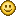#### 2DIndie

Perfect!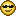Thx!